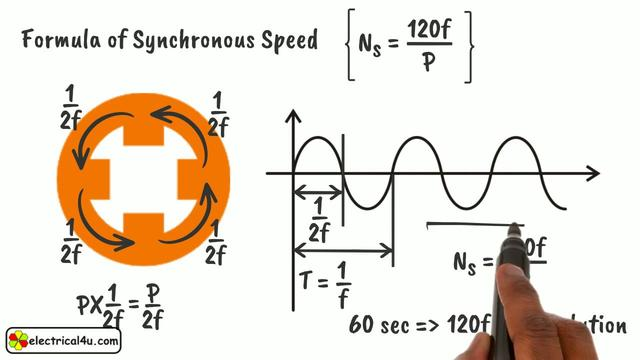# Synchronous speed

## Calculation formula

When the rotating magnetic field has opposite poles, the magnetic field will rotate in space every time the alternating current changes. So the synchronous speed is

where:

——the frequency of the current;——the number of magnetic pole pairs produced by the stator winding .

## Application

The frequency (ie power frequency) of the power supply current of my country's power grid is (that is, the change of 50 cycles per second), then the speed of a pair of rotating magnetic fields is 50r/min ×60=3000 r/min.

If the stator windings are arranged differently, the number of magnetic pole pairs produced is also different, as shown in the motor shown in Figure 1. If the two windings on the stator that are separated by 180° are connected in series as a phase winding (for example, the U-phase winding is composed of windings and series), and finally the three-phase windings are connected according to the star connection (or delta connection) When a three-phase power supply is used, a rotating magnetic field with two pairs of magnetic poles can be generated.

Whenever the alternating current changes for one cycle, the two-pole rotating magnetic field rotates through a 360° (1 revolution) mechanical angle in space. The four-pole rotating magnetic field only rotates through a 180° (1/2 turn) mechanical angle in space during one cycle of current change.

The speed of the motor, represented by n. The rotor is driven by the rotating magnetic field to run. When the asynchronous motor is in the electric state, its speed is always less than the synchronous speed n1. This is because the rotor rotates in the same direction as the magnetic field, and the rotor rotates slower than the magnetic field. It is possible to cut the magnetic lines of force and generate induced currents, and the rotor can also be subjected to the action of magnetic torque.

If there is a situation, it means that there is no relative movement between the rotor and the magnetic field, and the rotor does not cut the magnetic lines of force, and no induced current will be generated in the rotor, and it will not receive the action of magnetic torque. If this happens, the rotor will gradually decelerate under the action of resistance torque (from friction or load), so that. When the electromagnetic torque and resistance torque (the sum of friction torque and load torque) on the rotor are balanced, the rotor keeps rotating at a constant speed. Therefore, when the asynchronous motor is running normally, this is the reason why this type of motor is called "asynchronous" motor. And because the current in the rotor is not supplied by the power supply, but generated by electromagnetic induction, this type of motor is also called an induction motor.

Related Articles